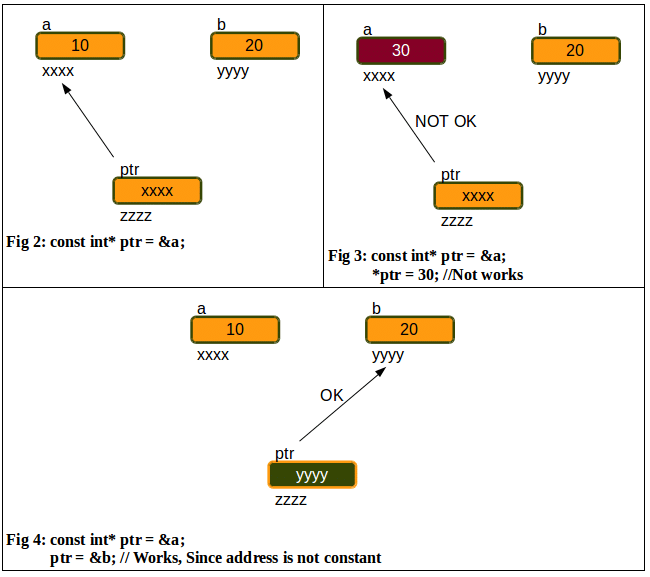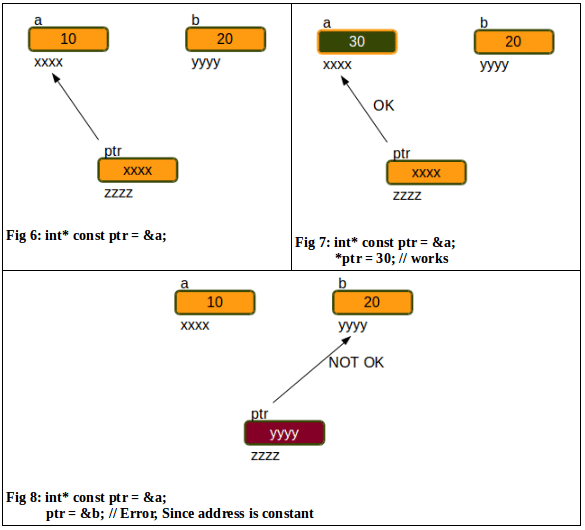# Constant Pointers and Pointers to Constant A Subtle Difference in C Programming

1
14185Pointers have always been a complex topic to understand for those new to C programming. There will be more confusion for newbies when these terms are used along with some qualifiers like const in C programming. In this article, I will focus on the difference between the pointers to constant and constant pointers in order to make the concepts very clear.

Note: The code snippets provided here have been tested with the GCC compiler [gcc version 4.8.2] running under the Linux environment.

Pointer to constant
As the name itself indicates, the value of the variable to which the pointer is pointing, is constant. In other words, a pointer through which one cannot change the value of the variable to which it points is known as a pointer to constant.

Note : These pointers can change the address they point to but cannot change the value at the address they are pointing to.

Illustration 1
Let us consider the code snippet given below to understand how pointer to constant works:

```1 #include <stdio.h>
2
3 int main()
4 {
5 //Definition of the variable
6 int a = 10;
7
8 //Definition of pointer to constant
9 const int* ptr = &a; //Now, ptr is pointing to the value of the variable a
10
11 *ptr = 30; //Error: Since the value is constant
12
13 return 0;
14 }```

In the above code, in Line No. 11, we are trying to change the value of the variable to which the pointer is pointing to, but this is not possible since the value is constant. When the above code is compiled and run, we get the output shown in Figure 1.

Illustration 2
Now, lets use the same example given in Illustration 1 to show that the address that the pointer contains is not a constant.

```1 #include <stdio.h>
2
3 int main()
4 {
5 //Definition of the variables
6 int a = 10;
7 int b = 20;
8
9 //Definition of pointer to constant
10 const int* ptr = &a; //Now, ptr is pointing to the value of the variable a
11
12 ptr = &b; // Works: Since pointer is not constant
13
14 return 0;
16 }```

From Illustrations 1 and 2, one can understand that the address that the pointer contains can be changed but not the value to which the pointer is pointing to. This can be clearly understood by the pictorial representations given in Figures 2, 3 and 4.Constant pointers
Aconstant pointer is one that cannot change the address it contains. In other words, we can say that once a constant pointer points to a variable, it cannot point to any other variable.
Note: However, these pointers can change the value of the variable they point to but cannot change the address they are holding.

Illustration 3
Let us consider the following code snippet to understand howconstant pointer works:

```1 #include <stdio.h>
2
3 int main()
4 {
5 //Definition of the variable
6 int a = 10;
7 int b = 20;
8
9 //Definition of pointer to constant
10 const int* ptr = &a;//Now, ptr is pointing to the value of the variable a
11
12 *ptr = 30; // Works,since the pointer pointing to the value is not constant
13
14 ptr = &b; //Error:Now, ptr is pointing to the value of the variable b
15
16 return 0;
17
18 }```From the above example (Illustration 3), it is clear that in Line No 14 we tried to change the address of the pointer ptr to some other variable, but it is not possible. The output of the code snippet shown in Illustration 3 is given in Figure 5. Similarly, one can observe that in Line No 12, we are trying to change the value of the variable it is pointing to, which is possible.
This can be clearly understood by the pictorial representations given in Figures 6, 7 and 8.

 Something to think about: Can we have both pointer to constant and constant pointer in a single statement?

Usage
We can find n number of uses of these concepts in C as well as in the embedded C programming world. One such simple use of pointer to constant is to find the string length of the given string without any attempt to modify the original string as shown in Example 1 (Figure 9). Example 2 gives an idea of using pointer to constant in the strcmp() function (Figure 10).

A trick
There is a small trick to understand the difference between pointer to constant and constant pointers which is shown in Table 6.
Note: This trick is for all those new to the C programming world, who are confused with constant and pointers.

From the summary shown in Table 5, separate the part before asterisk(*) and the part after the asterisk(*) as given in Table 6, to clearly understand whether data is constant or pointer is constant.#### 1 COMMENT

1.costel0103

Regarding Illustration 3 :

const int* ptr = &a; => As long as the illustration is about constant pointer, shoudn’t it be “int * const ptr” ?